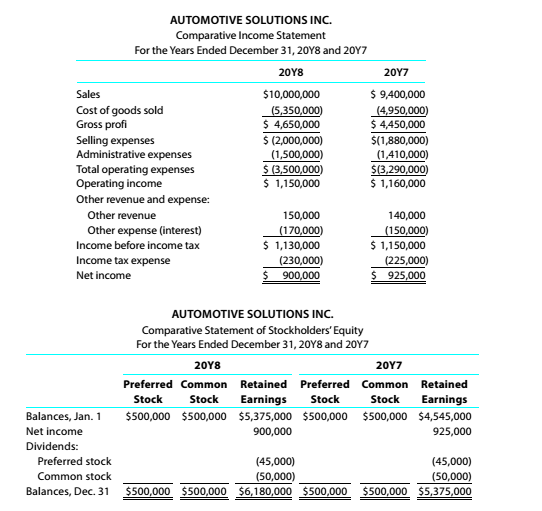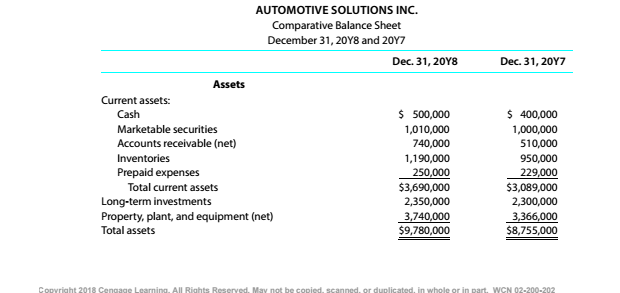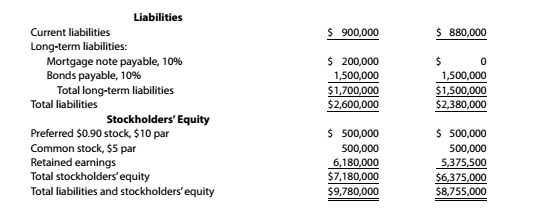Chapter 9, Problem 9.4.19P

Chapter
Section
Textbook Problem

Twenty metrics of liquidity, solvency, and profitabilityThe comparative financial statements of Automotive Solutions Inc. are as follows. The market price of Automotive Solutions Inc. common stock was \$119.70 on December 31, 20Y8InstructionsDividends per share of common stock

To determine

Concept Introduction:

Dividend Yield Ratio:

Dividend Yield ratio is calculated as percentage by dividing the Dividend per share by Market price per share. The formula for the Dividend Yield ratio is as follows:

Dividend Yield Ratio =  Dividend per shareMarketprice per share

To Calculate:

The dividend per share of common stock.

Explanation

The Dividend per Share of Common Stock is calculated as follows:

 20Y8 Common Dividend Paid (A)

Still sussing out bartleby?

Check out a sample textbook solution.

See a sample solution

The Solution to Your Study Problems

Bartleby provides explanations to thousands of textbook problems written by our experts, many with advanced degrees!

Get Started